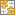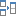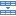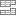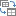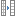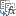# How to get absolute value in Excel: ABS function with formula examples

The tutorial explains the concept of the absolute value of a number and shows some practical applications of the ABS function to calculate absolute values in Excel: sum, average, find max/min absolute value in a dataset.

One of the fundamental things we know about numbers is that they can be positive and negative. But sometimes you may need to use only positive numbers, and that's where the absolute value comes in handy.

## Absolute value of a number

In simple terms, the absolute value of a number is the distance of that number from zero on a number line, regardless of the direction.

For example, the absolute value of the number 3 and -3 is the same (3) because they are equally far from zero: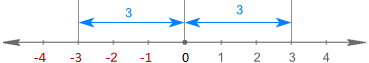From the above visual, you can figure out that:

• The absolute value of a positive number is the number itself.
• The absolute value of a negative number is the number without its negative sign.
• The absolute value of zero is 0.

Easy!

In math, the absolute value of x is denoted as |x| like in these examples:

`|-10| = 10           |10| = 10`

In Excel, there is no absolute value symbol, but there is a special function for getting an absolute value - ABS function.

Note. Excel absolute value should not be confused with absolute cell reference. The latter is a special form of a cell address that locks a reference to a given cell. For more information, please see Absolute cell references in Excel.

## ABS function in Excel

The ABS function in Excel has just one purpose - to get the absolute value of a number.

ABS(number)

Where number is the number you want to get the absolute value of. It can be represented by a value, cell reference or another formula.

For example, to find the absolute value of a number in cell A2, you use this formula:

`=ABS(A2)`

The following screenshot shows our absolute formula in Excel: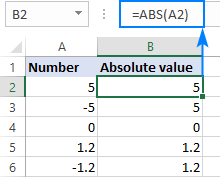## How to calculate absolute value in Excel

You now know the concept of absolute value and how to calculate it in Excel. But can you think of real-life applications of an absolute formula? The following examples will hopefully help you gain a better understanding of what you are really finding.

### Convert negative numbers to positive numbers

In situations when you need to change negative number to positive numbers, the Excel ABS function is an easy solution.

Supposing, you calculate the difference between two numbers by subtracting one number from the other. The problem is that some of the results are negative numbers while you want the difference to always be a positive number: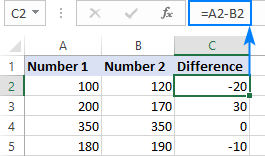Wrap the formula in the ABS function:
`=ABS(A2-B2)`

And have the negative numbers converted to positive, leaving the positive numbers unaffected: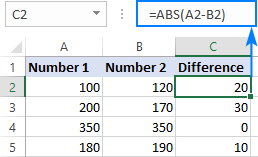### Find if value is within tolerance

Another common application of the ABS function in Excel is to find whether a given value (number or percentage) is within expected tolerance or not.

With the actual value in A2, expected value in B2, and the tolerance in C2, you build the formula in this way:

• Subtract the expected value from the actual value (or the other way round) and get the absolute value of the difference: ABS(A2-B2)
• Check if the absolute value is less than or equal to the allowed tolerance: ABS(A2-B2)<=C2
• Use the IF statement to return the desired messages. In this example, we return "Yes" if the difference is within tolerance, "No" otherwise:

`=IF(ABS(A2-B2)<=C2, "Yes", "No")`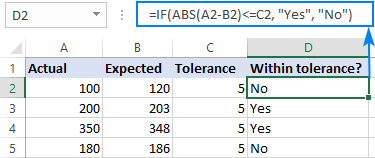### How to sum absolute values in Excel

To get an absolute sum of all numbers in a range, use one of the following formulas:

Array formula:

SUM(ABS(range))

Regular formula:

SUMPRODUCT(ABS(range))

In the first case, you use an array formula to force the SUM function to add up all the numbers in the specified range. SUMPRODUCT is an array type function by nature and can handle a range without extra manipulations.

With the numbers to be summed in cells A2:B5, either of the following formulas will work a treat:

Array formula, completed by pressing Ctrl + Shift + Enter:

`=SUM(ABS(A2:B5))`

Regular formula, completed with a usual Enter keystroke:

`=SUMPRODUCT(ABS(A2:B5))`

As shown in the screenshot below, both formulas sum absolute values of positive and negative numbers, ignoring the sign: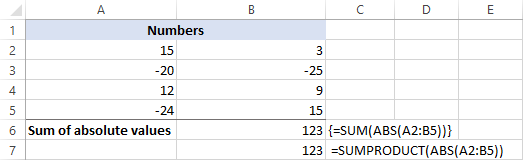### How to find the maximum/minimum absolute value

The easiest way to get the minimum and maximum absolute value in Excel is to use the following array formulas.

Maximum absolute value:

MAX(ABS(range))

Minimum absolute value:

MIN(ABS(range))

With our sample dataset in A2:B5, the formulas take the following shape:

To get the max absolute value:

`=MAX(ABS(A2:B5))`

To find the min absolute value:

`=MIN(ABS(A2:B5))`

Please be sure to properly complete the array formulas by pressing Ctrl+Shift+Enter.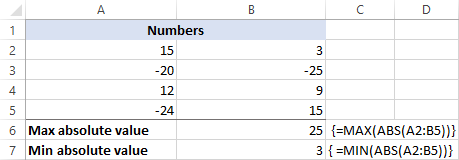If you don't like using array formulas in your worksheets, you can trick the ABS function into processing a range by nesting it into the array argument of the INDEX function like shown below.

To get the maximum absolute value:

`=MAX(INDEX(ABS(A2:B5),0,0))`

To get the minimum absolute value:

`=MIN(INDEX(ABS(A2:B5),0,0))`

This works because an INDEX formula with the row_num and column_num arguments set to 0 or omitted tells Excel to return a whole array rather than an individua value.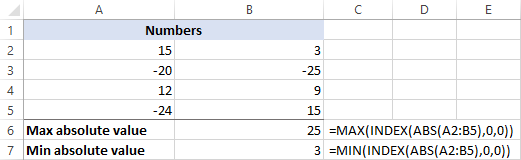### How to average absolute values in Excel

The formulas we used to calculate the min/max absolute value can average absolute values too. You will just have to replace MAX/MIN with the AVERAGE function:

Array formula:
`=MAX(ABS(range))`

Regular formula:
`=AVERAGE(INDEX(ABS(range),0,0))`

For our sample data set, the formulas would go as follows:

Array formula to average absolute values (entered by pressing Ctrl + Shift + Enter):
`=MAX(ABS(A2:B5))`

Regular formula to average absolute values:
`=AVERAGE(INDEX(ABS(A2:B5),0,0))`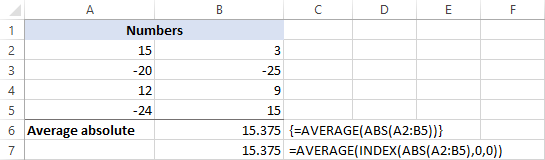## More absolute value formula examples

Apart from the typical uses of an absolute value demonstrated above, the Excel ABS function can be used in combination with other functions to handle the tasks for which there is no built-in solution. Below you can find a few examples of such formulas.

Get a date closest to today - an absolute value is used to get a date nearest to today.

Calculate rank by absolute value - rank numbers by their absolute values ignoring the sign.

Extract a decimal part of a number - get a fractional part of a number as an absolute value.

Get a square root of a negative number - take a square root of a negative number as if it were a positive number.

That's how to do absolute value in Excel by using the ABS function. The formulas discussed in this tutorial are very straightforward and you will hardly have any difficulties adjusting them for your worksheets. To have a closer look, you're welcome to download our sample Excel Absolute Value workbook.

I thank you for reading and hope to see you on our blog next week!

### 5 responses to "How to get absolute value in Excel: ABS function with formula examples"

1.Sailesh says:

wow nice teaching
thanks for this.

2.arjun says:

hi, i wand excel code for below mentioned control
if -0.040 below and 0.040 above will come red color in excel sheet cell
For example i get value -0.050 need red color and same cell i get value 0.050 need red color.
need for your guidance as soon as possible.

3.Juvit says:

I want to retain the negative sign on the found maximum..how?

4.Arjun Suresh Kachrare says:

Can we use ABS function in multiple sheet in excel

•Alexander Trifuntov (Ablebits.com Team) says:

Hello Arjun !